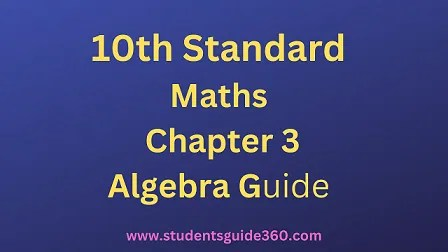# 10th Maths Chapter 3 Algebra Exercise 3.19

10th Standard Maths Chapter 3 Algebra Samacheer Kalvi Guide Exercise 3.19 Book Back Answers Solutions. TN 10th SSLC Samacheer Kalvi Guide. 10th All Subject Guide – Click Here. Class 1 to 12 All Subject Book Back Answers – Click Here## TN 10th STD Maths – Chapter 3 – Algebra – Ex 3.19

### 1. Find the order of the product matrix AB ifSolution:### 2. If A is of order p × q and B is of order q × r what is the order of AB and BA?

Solution:
If A is of order p × q [∵ p × q q × r = p × r]
the order of AB = p × r [∵ q × r p × q = r ≠ p]
Product of BA cannot be defined/found as the number of columns in B ≠. The number of rows in A.

### 2. If A is of order p × q and B is of order q × r what is the order of AB and BA?

Order of A = a × (a + 3)
Order of B = b × (17 – b)
Given: Product of AB exist
a + 3 = b
a – b = – 3 ….(1)
Product of BA exist
17 – b = a
– a – b = -17
a + b = 17 ………(2)
(1) + (2) ⇒ 2a = 14
a = 142 = 7
Substitute the value of a = 7 in (1)
7 – b = -3 ⇒ -b = -3 -7
-b = -10 ⇒ b = 10
The value of b = 7 and b = 10

###Solution:5.Solution:### Solution:7.(i) A(BC) = (AB)C
(ii) (A – B)C = (AC – BC)
(iii) (A- B)T = AT – BT
Solution:
(i) A(BC) = (AB)C8.Solution:9.### 10. Verify that A2 = I when A = (56−4−5)

Solution:11.12.Solution:### 13. If A =  show that A2 – 5A + 7I2 = 0.

Solution: